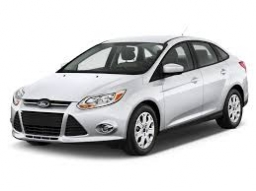# Deceleration

In a braking test, a vehicle that travels at 51 km/h stops in 5 s. What is its acceleration?

a =  -2.8333 m/s2

### Step-by-step explanation:Did you find an error or inaccuracy? Feel free to write us. Thank you!

Tips for related online calculators
Need help calculating sum, simplifying, or multiplying fractions? Try our fraction calculator.
Do you know the volume and unit volume, and want to convert volume units?
Do you want to convert velocity (speed) units?
Do you want to convert time units like minutes to seconds?